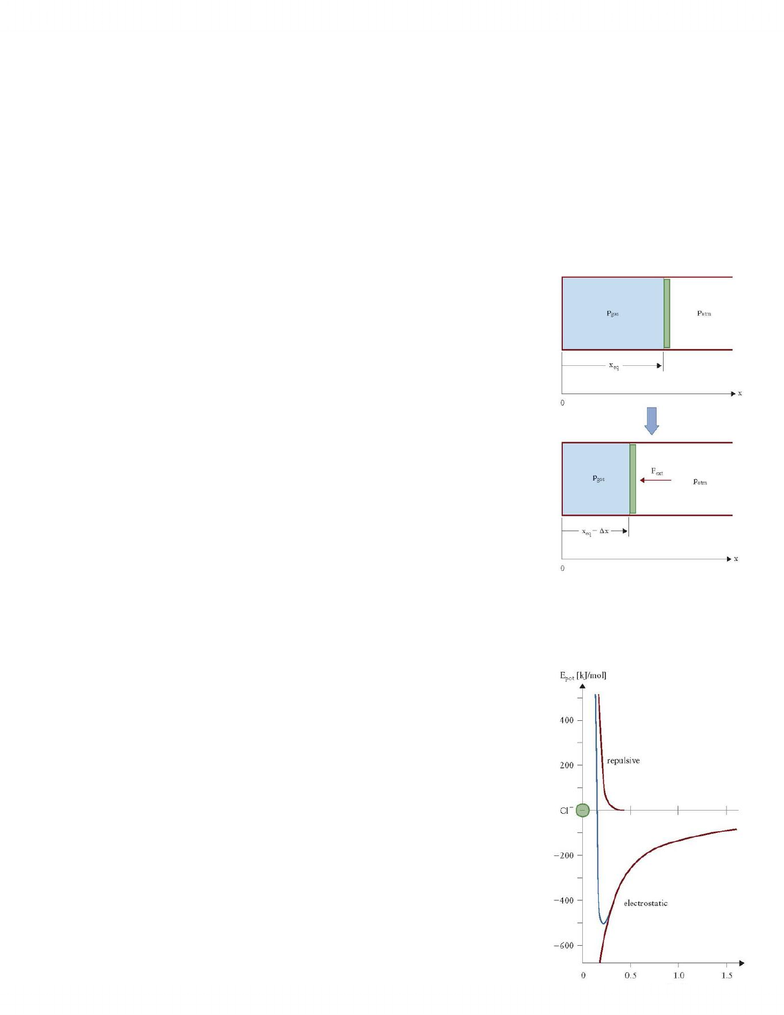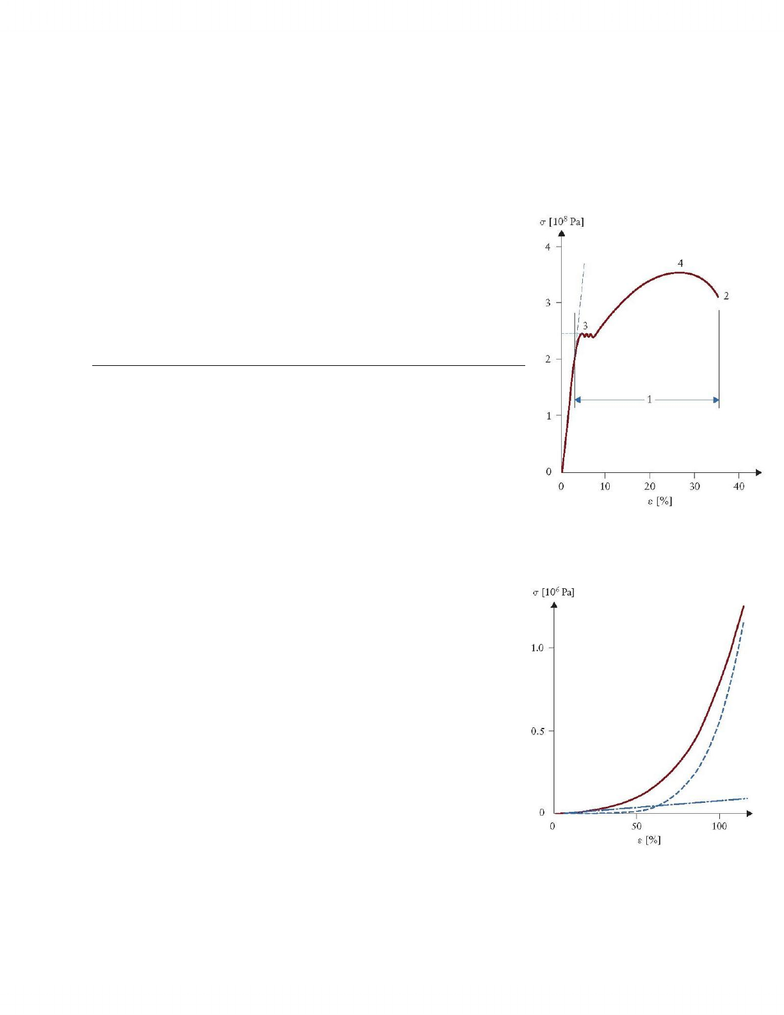# Physics 1021 Study Guide - Quiz Guide: Electric Dipole Moment, Electric Potential Energy, Mechanical Equilibrium

23 views20 pages
School
Western University
Department
Physics
Course
Physics 1021RM QUIZ 14 AND 15
(MC-12-WN-001) An object is attached to a horizontal spring. It is initially displaced by a given distance
from the equilibrium position (index 1). If I double the displacement (index 2), the elastic energy of the
object changes from E1 to E2, where:
A. ... E2 = E1, i.e., energy is conserved
B. ... E2 = 0.5 E1
C. ... E2 = 2 E1
D. ... E2 = 0.25 E1
E. ... E2 = 4 E1
(MC-12-WN-002) An object is attached to a horizontal spring. It is initially displaced by distance Δx from
the equilibrium position and then released from rest. At what point of its motion along the x-axis
reaches the object its highest potential energy?
A. At the equilibrium position
B. At the position where it is released
C. At midway between the amplitude and equilibrium positions
D. The potential energy is the same at all positions
E. The object reaches its highest potential energy only during a later cycle, not during the first oscillation
(MC-12-WN-003) An object is attached to a horizontal spring. It is initially displaced by distance Δx from the
equilibrium position and then released from rest. At what point of its motion along the x-axis reaches the object its
highest total energy?
A. At the equilibrium position
B. At the position where it is released
C. At midway between the amplitude and equilibrium positions
D. The total energy is the same at all positions
E. The object reaches its highest total energy only during a later cycle, not during the first oscillation
(MC-12-WN-004) An object is attached to a horizontal spring. It is initially displaced by distance Δx from
the equilibrium position and then released from rest. When the object passes the equilibrium position,
its speed will be:
A. v = 0 m/s
B. proportional to Δx (the speed is proportional to the initial displacement)
C. proportional to Δx2 (speed is proportional to the square of the initial displacement)
D. is a non-linear function of the Δx, but cannot be written in the form Δv proportional to Δx2
Unlock document

This preview shows pages 1-3 of the document.
Unlock all 20 pages and 3 million more documents.(MC-12-WN-005) An object is attached to a horizontal spring. The equilibrium position of the object is at
position x = xeq. The elastic force due to the spring is written as: Felast = - k(x - xeq)
What does the negative sign in front of the bracket on the right hand side imply?
A. The object is in mechanical equilibrium
B. The elastic force is a restoring force
C. The object displays cyclic motion
D. The object displays a sinusoidal motion
E. The energy of the system is conserved
(MC-12-WN-006) The attached figure shows a mobile piston that seals a gas
in a horizontal cylinder. The piston is in mechanical equilibrium at position
xeq where the gas pressure pgas is equal to the external atmospheric
pressure patm. An external force allows us to move the piston to a new
position at xeq - x. The origin of the x-axis is chosen at the left end of the gas
cylinder. Which statement is incorrect regarding the lower part of the figure?
A. The piston is in mechanical equilibrium
B. When released (Fext removed) the piston travels toward smaller x-values
C. After release, an ideal (frictionless) piston will move back and forth in a
harmonic fashion
D. Immediately after the piston is released, the gas expands
E. Immediately after the piston is released, the gas does work on the piston
(MC-12-WN-007) Consider the elastic force equation: Felast = -k (x-xeq)
What does the negative sign in the bracket on the right hand side imply?
A. The force is proportional to the displacement
B. The equilibrium position lies at a positive x position
C. The object is located at the equilibrium position
D. The forces increases when the object moves to positive x-values
E. The energy of the system is conserved
(MC-12-WN-008) The attached figure shows the potential energy as a
function of distance between a Na+ ion and a Cl- ion in rock salt (NaCl). The
electrostatic attraction between the ions is the red curve at negative
energies, the repulsive energy is the red curve at positive energies. The
combined potential energy curve for the NaCl bond is shown as an
asymmetric blue curve.
A. The blue line represents the potential energy curve for a harmonic
oscillator
B. The red line labelled "electrostatic" represents the potential energy curve
Unlock document

This preview shows pages 1-3 of the document.
Unlock all 20 pages and 3 million more documents.for a harmonic oscillator
C. The red line labelled "replusive" represents the potential energy curve for a harmonic oscillator
D. Only the lower part of the blue curve can be modeled (approximately) as the potential energy of a
harmonic oscillator
E. The harmonic oscillator model cannot be used to describe the NaCl bond in rock salt
(MC-12-WD-001) The attached figure shows the stress-strain relation for
steel. Up to which stress value does the strain in steel respond linearly to
the exerted stress? (Choose closest value)
A. σ = 0 Pa
B. ε = 30 %
C. σ = 3.5 x 108 Pa
D.
ε = 4 %
E. σ = 2 x 108 Pa
(MC-12-WD-002) Comparing the two attached figures, the following
statement is correct:
A. Both steel and blood vessels require roughly the same strain for a given
stress
B. Hooke's law can be applied to both systems
C. Due to the differences in stress for a given strain, steel is called a hard material and blood vessel
tissue is called a soft material
D. Steels ruptures at much larger strain values than blood vessel tissue
E. None of the statements is correct
(MC-12-WD-003) The attached figure shows the stress-strain relation for
the elastic tissue of blood vessels. The blood vessel contains two
components which play a role in its elastic properties: elastin (dash-dotted
curve) and collagen with a bulk modulus increasing with strain (dashed
curve). These contributions are combined (solid curve) for an actual blood
vessel. Based on the figure, which of the following statements is true?
A. The elastic properties of elastin cannot be described by Hooke's law
B. Collagen shows a linear stress-strain behaviour up to strains of 100%
C. Collagen shows a linear stress-strain behaviour up to strains of about
50%
D. The actual blood vessel wall shows no linear stress-strain behaviour
due to its collagen component
E. The actual blood vessel wall shows no linear stress-strain behaviour due to its elastin component
Unlock document

This preview shows pages 1-3 of the document.
Unlock all 20 pages and 3 million more documents.

## Document Summary

Rm quiz 14 and 15 (mc-12-wn-001) an object is attached to a horizontal spring. It is initially displaced by a given distance from the equilibrium position (index 1). It is initially displaced by distance x from the equilibrium position and then released from rest. The equilibrium position of the object is at position x = xeq. The elastic force due to the spring is written as: felast = - k(x - xeq) The piston is in mechanical equilibrium at position xeq where the gas pressure pgas is equal to the external atmospheric pressure patm. An external force allows us to move the piston to a new position at xeq - x. The origin of the x-axis is chosen at the left end of the gas cylinder. The electrostatic attraction between the ions is the red curve at negative energies, the repulsive energy is the red curve at positive energies.